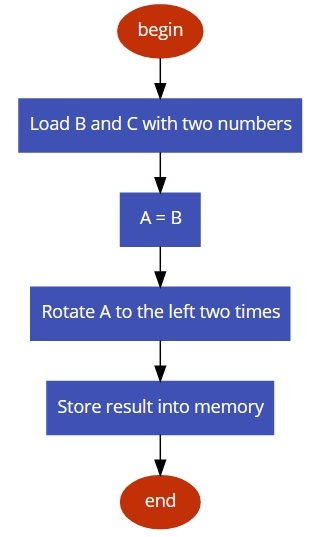# 8085 program to multiply two 8 bit numbers using logical instructions

In this program we will see how to multiply using logical operators.

## Problem Statement

Write 8085 Assembly language program to multiply two 8-bit numbers using logical operators.

## Discussion

We are assuming the first number is in register B, and second number is in register C, and the result must not have any carry.

Here we are multiplying with 04H. We can perform the multiplication by left rotate two times. Assign 06H into B, and 04H into C. Load B to A, then rotate the accumulator two times. Store the result into a specified memory.

Register
Data
B
06
C
04

## Flow Diagram## Program

HEX Codes
Labels
Mnemonics
F000
06, 06

MVI B,06H

F002
0E, 04

MVI C,04H

F004
78

MOV A,B
F005
07

RLC
Rotate left without carry
F006
07

RLC
Rotate left without carry
F007
32, 00, 80

STA 8000H
Store result into 8000H
F00A
76

HLT
Terminate the program